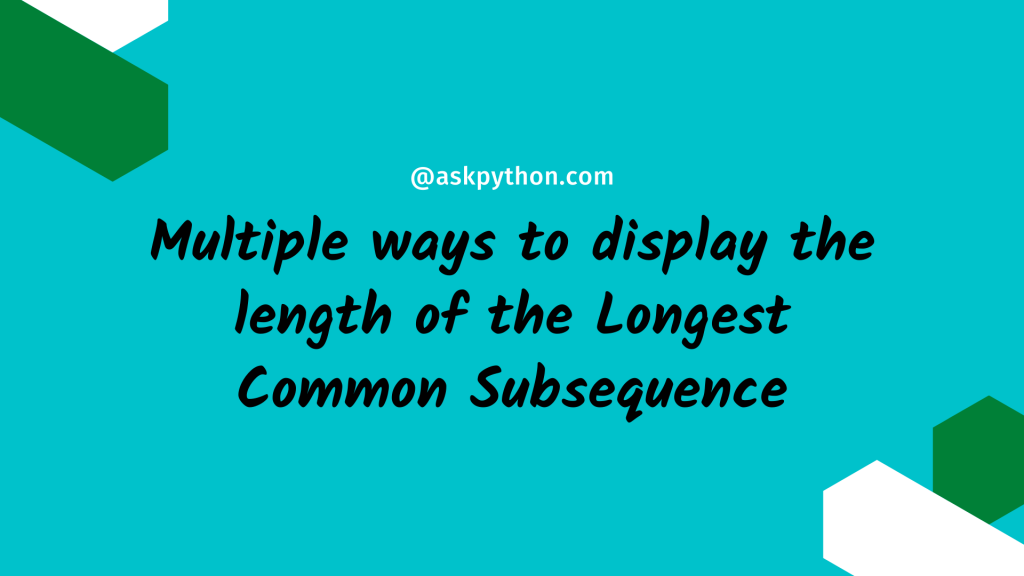# Find The Length of the Longest Common SubsequenceIn this tutorial, we will first briefly explain what subsequence and longest common subsequence are before diving right into the code. In the code part, we will learn how to use recursion and dynamic programming to discover the length of the longest common subsequence.

Let’s get started right away.

## What is a Subsequence?

A string subsequence is a new string created by removing part of the characters from the previous string while leaving the relative positions of the characters unaltered.

As an example-
Original string = “ABCDVWXYZ”
Valid subsequences = “ACDW”, ”BYZ”, ”ACWXYZ”
Invalid subsequences = “VAYZ”, “DYAZ”, “XBACW”

## What is Longest Common Subsequence (LCS)?

Given a set of sequences, the largest common subsequence challenge is to identify the longest subsequence shared by all of the sequences. The answer to the longest common subsequence issue is not always unique. There may be many common subsequences with the longest feasible length.

As an example-
Sequence1 = “BAHJDGSTAH”
Sequence2 = “HDSABTGHD”
Sequence3 = “ABTH”
Length of LCS = 3
LCS = “ATH”, “BTH”

## Method 1: Recursion

We begin comparing the strings from the end, one character at a time, in recursion. Let LCS be the function for determining the length of the longest subsequence shared by two strings.

There are two probable scenarios:

1. Characters are the same – add 1 to LCS and execute the procedure recursively using the updated strings by eliminating the last characters – LCS (str1, str2, m-1, n-1).
2. Characters are distinct – No more than (recursive call with sring 1 with the last character removed, recursive call with string 2 with the last character removed).
```def lcs(str1, str2, m, n):
if m==0 or n==0:
return 0
elif str1[m-1] == str2[n-1]:
return 1+lcs(str1, str2, m-1, n-1)
else:
return max(lcs(str1, str2, m-1, n),lcs(str1, str2, m,n-1))
str1 = input("Enter first string: ")
str2 = input("Enter second string: ")
lcs_length = lcs(str1, str2, len(str1), len(str2))
print("length of LCS is : {}".format(lcs_length))
```
```Enter first string: BAHJDGSTAH
Enter second string: BAHJDGSTAH
length of LCS is : 5
```

## Method 2: Dynamic Programming Appraoch

The bottom-up strategy is used in this technique. The subproblem solutions are saved in a matrix for future use. This is referred to as memoization. If the lengths of two strings are m and n, respectively, the time complexity of dynamic programming is O(mn), which is substantially less than the time complexity of recursion. The matrix’s last entry represents the length of the LCS.

```def lcs(str1 , str2):
m = len(str1)
n = len(str2)
matrix = [*(n+1) for i in range(m+1)]
for i in range(m+1):
for j in range(n+1):
if i==0 or j==0:
matrix[i][j] = 0
elif str1[i-1] == str2[j-1]:
matrix[i][j] = 1 + matrix[i-1][j-1]
else:
matrix[i][j] = max(matrix[i-1][j] , matrix[i][j-1])
return matrix[-1][-1]
str1 = input("Enter first string: ")
str2 = input("Enter second string: ")
lcs_length = lcs(str1, str2)
print("Length of LCS is : {}".format(lcs_length))
```
```Enter first string: BAHJDGSTAH
Enter second string: BAHJDGSTAH
length of LCS is : 5
```

## Conclusion

Congratulations! You just learned how to display the length of the Longest Common Subsequence.

Liked the tutorial? In any case, I would recommend you to have a look at the tutorials mentioned below:

Thank you for taking your time out! Hope you learned something new!! 😄